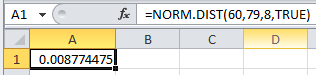# The time needed to complete a final examination in a particular college course is normally distributed with a mean of 79 minutes and a standard deviation of 8 minutes. Answer the following questions.a. What is the probability of completing the exam in one hour or less (to 4 decimals)?b. What is the probability that a student will complete the exam in more than minutes but less than minutes (to 4 decimals)?c. Assume that the class has students and that the examination period is minutes in length. How many students do you expect will be unable to complete the exam in the allotted time (to nearest whole number)?

Question
80 views

The time needed to complete a final examination in a particular college course is normally distributed with a mean of 79 minutes and a standard deviation of 8 minutes. Answer the following questions.

a. What is the probability of completing the exam in one hour or less (to 4 decimals)?

b. What is the probability that a student will complete the exam in more than minutes but less than minutes (to 4 decimals)?

c. Assume that the class has students and that the examination period is minutes in length. How many students do you expect will be unable to complete the exam in the allotted time (to nearest whole number)?
check_circle

Step 1

It is given that the time needed to complete the final exam is normally distributed with mean 79 minutes and standard deviation is 8 minutes.

Step 2

a).

Probability that completing the exam in one hour (60 minutes) or less can be obtained by entering the formula “=NORM.DIST(60,79,8,TRUE)” in EXCEL....

### Want to see the full answer?

See Solution

#### Want to see this answer and more?

Solutions are written by subject experts who are available 24/7. Questions are typically answered within 1 hour.*

See Solution
*Response times may vary by subject and question.
Tagged in

### Statistics